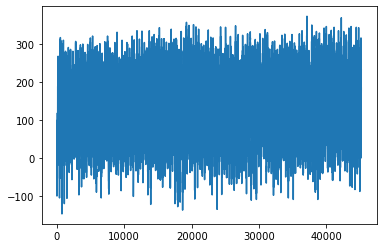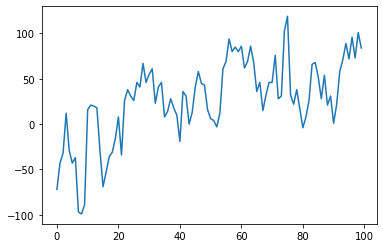# NumPy arrays¶

Nikolay Koldunov

[email protected]

================• a powerful N-dimensional array object
• tools for integrating C/C++ and Fortran code
• useful linear algebra, Fourier transform, and random number capabilities
In :
import numpy as np
%matplotlib inline

In :
np.set_printoptions(precision=3 , suppress= True) # this is just to make the output look better


Load data in to a variable:

In :
temp = np.loadtxt('Ham_3column.txt')

In :
temp

Out:
array([[1891.,    1.,    1.,  -72.],
[1891.,    1.,    2.,  -43.],
[1891.,    1.,    3.,  -32.],
...,
[2014.,    8.,   29.,  216.],
[2014.,    8.,   30.,  198.],
[2014.,    8.,   31.,  184.]])
In :
temp.shape

Out:
(45168, 4)In :
temp.size

Out:
180672

So it's a row-major order. Matlab and Fortran use column-major order for arrays.

In :
type(temp)

Out:
numpy.ndarray

Numpy arrays are statically typed, which allow faster operations

In :
temp.dtype

Out:
dtype('float64')

You can't assign value of different type to element of the numpy array:

In :
temp[0,0] = 'Year'

---------------------------------------------------------------------------
ValueError                                Traceback (most recent call last)
<ipython-input-9-c2dbfa0bc312> in <module>
----> 1 temp[0,0] = 'Year'

ValueError: could not convert string to float: 'Year'

Slicing works similarly to Matlab:

In :
temp[0:5,:]

Out:
array([[1891.,    1.,    1.,  -72.],
[1891.,    1.,    2.,  -43.],
[1891.,    1.,    3.,  -32.],
[1891.,    1.,    4.,   12.],
[1891.,    1.,    5.,  -29.]])
In :
temp[-5:-1,:]

Out:
array([[2014.,    8.,   27.,  219.],
[2014.,    8.,   28.,  234.],
[2014.,    8.,   29.,  216.],
[2014.,    8.,   30.,  198.]])

One can look at the data. This is done by matplotlib module:

In :
import matplotlib.pylab as plt
plt.plot(temp[:,3])

Out:
[<matplotlib.lines.Line2D at 0x11b17be10>]## Index slicing¶

In general it is similar to Matlab

First 12 elements of second column (months). Remember that indexing starts with 0:

In :
temp[0:12,2]

Out:
array([ 1.,  2.,  3.,  4.,  5.,  6.,  7.,  8.,  9., 10., 11., 12.])

First raw:

In :
temp[0,:]

Out:
array([1891.,    1.,    1.,  -72.])

## Exercise¶

• Plot only first 1000 values
• Plot last 1000 values
In [ ]:


In [ ]:


In [ ]:



We can create mask, selecting all raws where values in third raw (days) equals 10:

In :
mask = (temp[:,2]==10)


Here we apply this mask and show only first 5 raws of the array:

In :
temp[mask][:20,:]

Out:
array([[1891.,    1.,   10.,  -89.],
[1891.,    2.,   10.,  -19.],
[1891.,    3.,   10.,   32.],
[1891.,    4.,   10.,   84.],
[1891.,    5.,   10.,  188.],
[1891.,    6.,   10.,  130.],
[1891.,    7.,   10.,  161.],
[1891.,    8.,   10.,  171.],
[1891.,    9.,   10.,  221.],
[1891.,   10.,   10.,  181.],
[1891.,   11.,   10.,   80.],
[1891.,   12.,   10.,  107.],
[1892.,    1.,   10.,   -4.],
[1892.,    2.,   10.,   36.],
[1892.,    3.,   10.,   16.],
[1892.,    4.,   10.,  146.],
[1892.,    5.,   10.,  195.],
[1892.,    6.,   10.,  205.],
[1892.,    7.,   10.,  209.],
[1892.,    8.,   10.,  155.]])

You don't have to create separate variable for mask, but apply it directly. Here instead of first five rows I show five last rows:

In :
temp[temp[:,2]==10][-5:,:]

Out:
array([[2014.,    4.,   10.,  116.],
[2014.,    5.,   10.,   27.],
[2014.,    6.,   10.,  300.],
[2014.,    7.,   10.,  277.],
[2014.,    8.,   10.,  259.]])

You can combine conditions. In this case we select days from 10 to 12 (only first 10 elements are shown):

In :
temp[(temp[:,2]>=10)&(temp[:,2]<=12)][0:10,:]

Out:
array([[1891.,    1.,   10.,  -89.],
[1891.,    1.,   11.,   16.],
[1891.,    1.,   12.,   21.],
[1891.,    2.,   10.,  -19.],
[1891.,    2.,   11.,   36.],
[1891.,    2.,   12.,   31.],
[1891.,    3.,   10.,   32.],
[1891.,    3.,   11.,   46.],
[1891.,    3.,   12.,   46.],
[1891.,    4.,   10.,   84.]])

## Exercise¶

Select only summer months
Select only first half of the year
In [ ]:



## Basic operations¶

Create example array from first 12 values of second column and perform some basic operations:

In :
days = temp[0:12,2]
days

Out:
array([ 1.,  2.,  3.,  4.,  5.,  6.,  7.,  8.,  9., 10., 11., 12.])
In :
days+10

Out:
array([11., 12., 13., 14., 15., 16., 17., 18., 19., 20., 21., 22.])
In :
days*20

Out:
array([ 20.,  40.,  60.,  80., 100., 120., 140., 160., 180., 200., 220.,
240.])
In :
days*days

Out:
array([  1.,   4.,   9.,  16.,  25.,  36.,  49.,  64.,  81., 100., 121.,
144.])

What's wrong with this figure?

In :
plt.plot(temp[:100,3])

Out:
[<matplotlib.lines.Line2D at 0x11bfaee50>]## Exercise¶

• Create new array that will contain only temperatures

• Convert temperature to deg C

• Convert all temperatures to deg F

In [ ]:



## Basic statistics¶

Create temp_values that will contain only data values:

In :
temp_values = temp[:,3]/10.
temp_values

Out:
array([-7.2, -4.3, -3.2, ..., 21.6, 19.8, 18.4])

Simple statistics:

In :
temp_values.min()

Out:
-14.6
In :
temp_values.max()

Out:
37.3
In :
temp_values.mean()

Out:
12.488779667020898
In :
temp_values.std()

Out:
8.05358769929934
In :
temp_values.sum()

Out:
564093.2

You can also use sum function:

In :
np.sum(temp_values)

Out:
564093.2

One can make operations on the subsets:

## Exercise¶

Calculate mean for first 1000 values of temperature
In [ ]:



## Saving data¶

You can save your data as a text file

In :
np.savetxt('temp_only_values.csv',temp[:, 3]/10., fmt='%.4f')


In :
!head temp_only_values.csv

-7.2000
-4.3000
-3.2000
1.2000
-2.9000
-4.3000
-3.7000
-9.7000
-9.9000
-8.9000


You can also save it as binary:

In :
f=open('temp_only_values.bin', 'w')
temp[:,3].tofile(f)
f.close()


## Exercises¶

• Select and plot only data for October
• Calculate monthly means for years from 1990 to 1999 and plot them